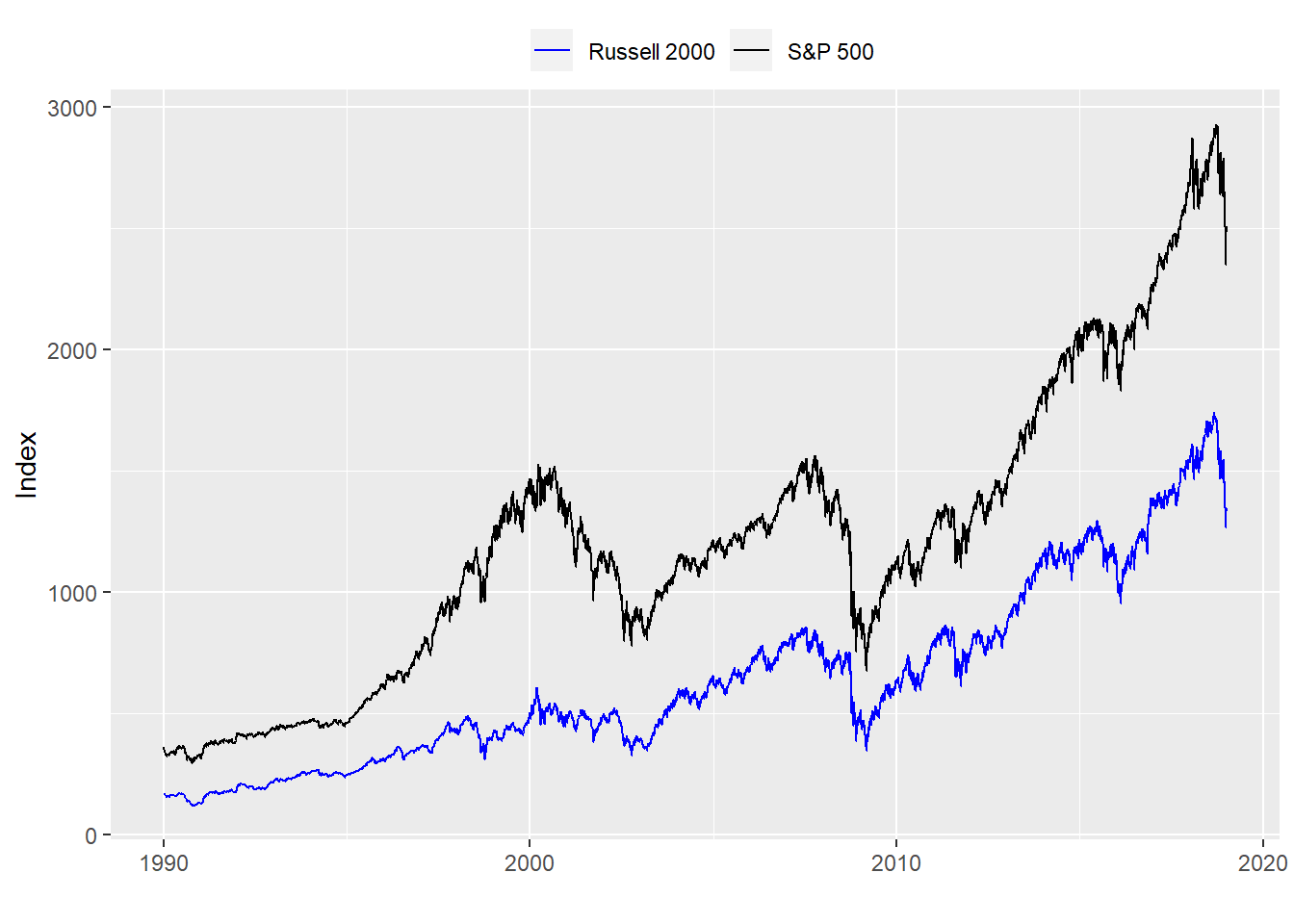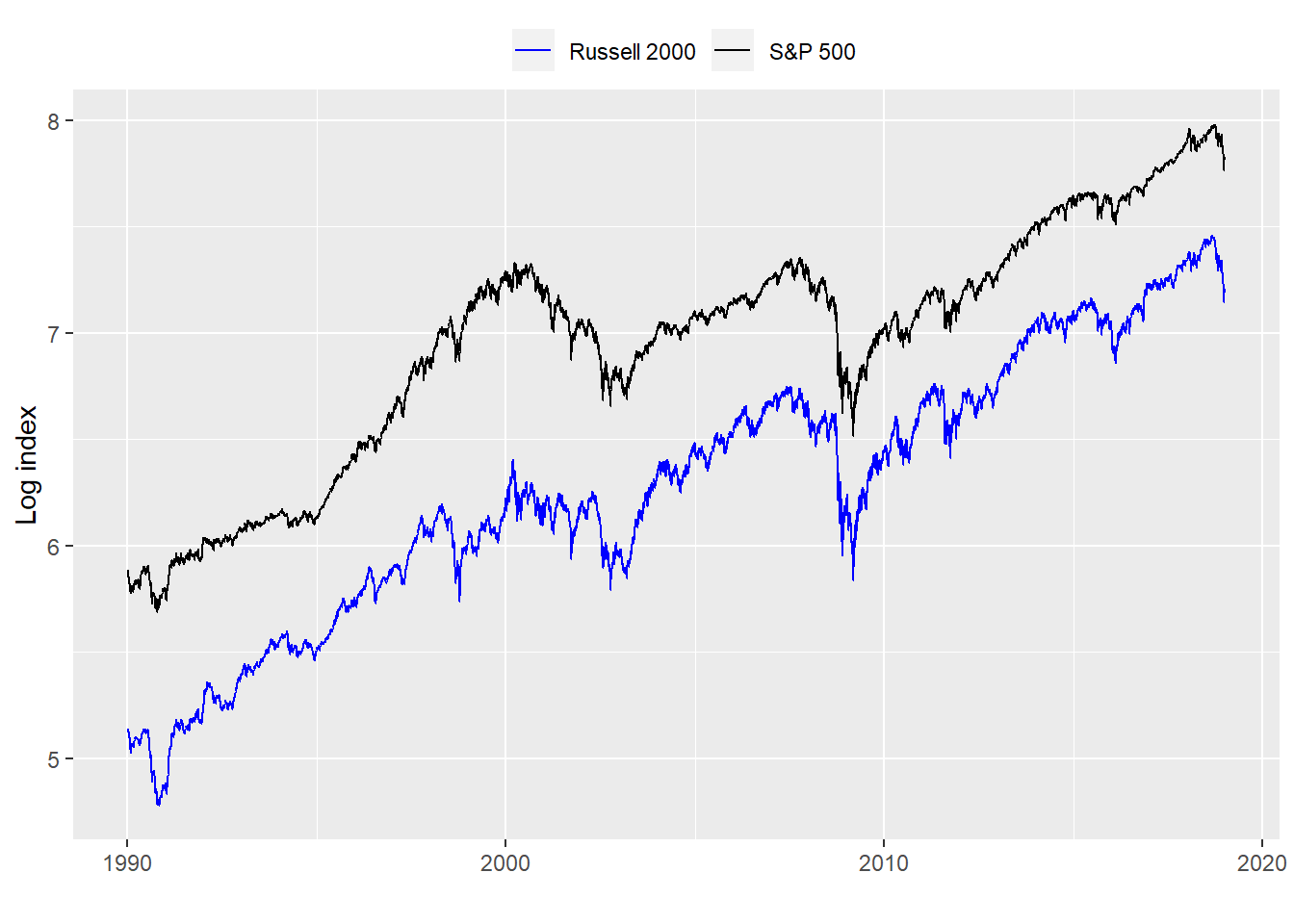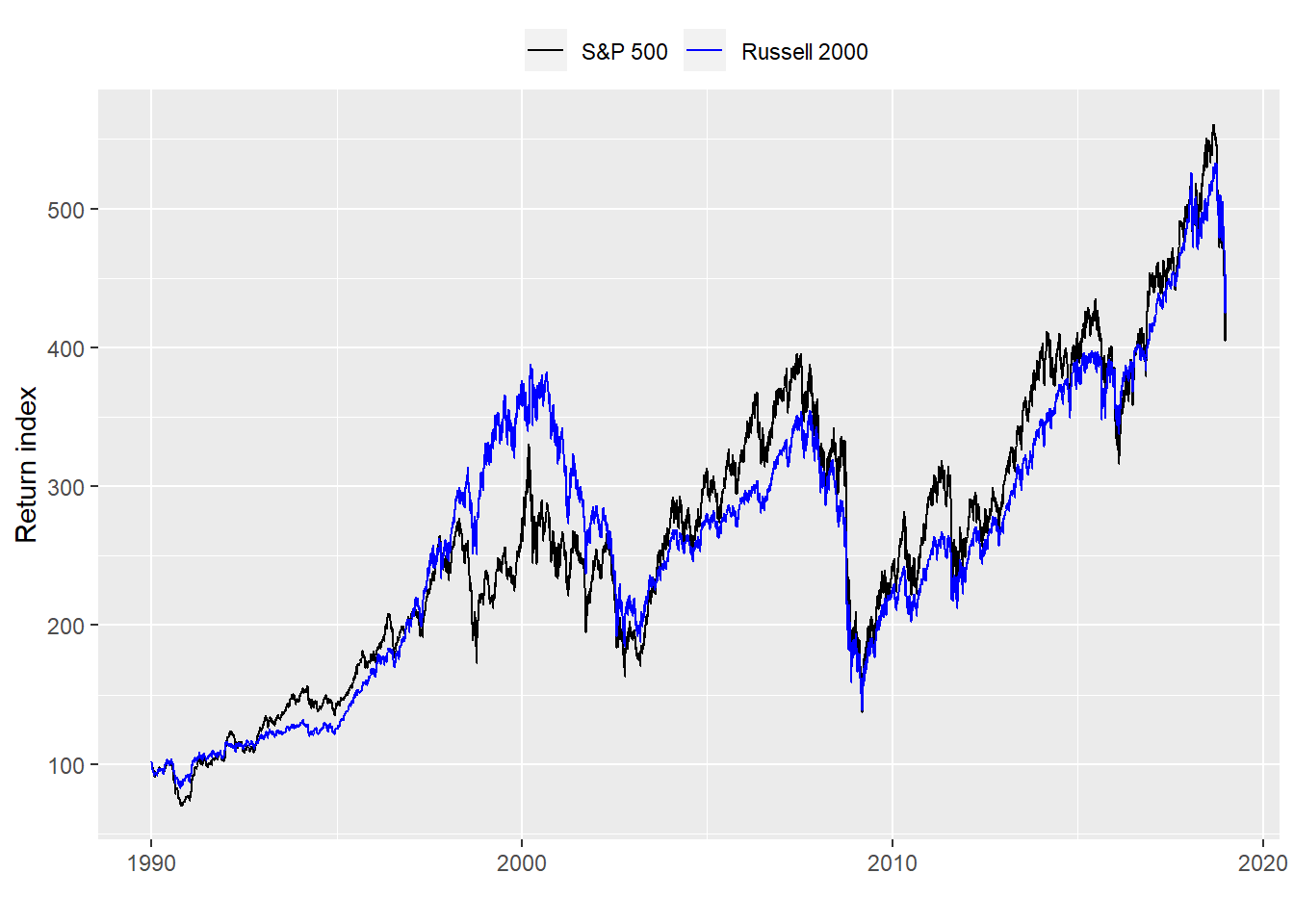# Stocks for some run

## Motivation

This string of posts is meant to help non-professional investors understand some of the complexities involved in choosing an investment strategy, suggest a logical framework on how to do just that, and offer different ways to analyze the data that motivate the strategies we’ll examine.

## Strategies for the long run

Investment strategies and styles abound — from fundamental to quantitative to technical. For the professional investor how much credence one gives to each approach depends as much on education, training, and employer as on open-mindedness and opportunity cost. For the non-professional, the opportunity cost to understand all the different strategies and to judge their merits is exceedingly high.

But what is an “investment strategy”? A working definition is that it is a plan to achieve a particular investment goal. Simple enough, but clearly one needs to understand the goal or goals before even evaluating the strategy. Yet most strategies cited in the press, online, or in blogs are often offered without much discussion of what the investment goal might be. The implicit assumption is probably something like, “maximize returns.” Yet comparing a strategy that invests in micro-cap stocks in the biotech sector to one that only invests in senior-secured debt of investment grade-rated companies using lots of leverage might produce similar returns. But the comparison, besides being a little goofy, is unlikely to be relevant without first understanding the investment goals.

So the first step is establishing a goal:

• For an individual that might be saving x dollars to live out retirement in comfort.
• For an insititution like a university it might be generating sufficient returns to support a proportional amount of the yearly operating or capital budget.

This is the “what” that the investor wants to achieve. Once the goal is determined, how it will be achieved tends to be based on weighing the merit of various investment “strategies”, like the small-cap vs corporate debt examples mentioned above. This is where things start to get complicated. You have an investment goal. Now you need to figure out which of the multiple strategies available (for example there are over 9,500 mutual funds in the US) are most likely to allow you to achieve that goal? How do you decide among the various strategies? How do you compare them? How do you know if they will work going forward?

## A modest strategy for evaluating strategies

One way to evaluate investing strategies is compare them to the most naive investmenting strategy possible. Prof. Rob Hyndman in Forecasting: Principles and Practice recommends a similar approach when comparing forecasting models. Establish a benchmark based on an average or naive forecast and then compare the more sophisticated forecast against that benchmark. In Hyndman’s suggestion a naive forecast would set all forecast data to the value of the last observation.

For investing, the most naive strategy out there (apart from stashing cash under a mattress) is probably “buy and hold” : just buy a stock (or any asset) and hold it forever (or least until you pass it on). But because it is naive, that doesn’t mean it’s bad. In fact, the hurdle for most strategies is to prove they beat buy and hold before or after transactions costs. Most strategies don’t. Or if they do, only the most sophisticated investors can implement them.

But there is a nuance here that might get missed. Comparing investing in large cap stocks to small cap stocks might seem straightforward. But what about comparing owning large cap stocks to a strategy that buys or sells large cap stocks based on some timing rules? What about comparing buying large cap stocks based on one set of timing rules vs. buying small cap stocks based on the same or different set of timing rules?

The first comparison is certainly about strategies. The historical record for risk and returns of large cap vs. small cap stocks might or might not be different. So if you’re investment goal has a return requirement (unlikely that it wouldn’t), your first step would be analyze the historical return record for competing strategies. Then you’d want to analyze risk and outlook. What about a benchmark? That becomes a bit more tricky. “Risk-free” government notes or securities might be one. But most educated folks are likely to realize that stocks enjoy higher returns than government bonds. So this comparison is trivial.

The second comparison is more about comparing tactics. Implcitly, you’ve committted yourself to the same exposure, unless, of course, the timing strategy meaningfully alters the risk/reward performance, which would be rare.

In the third example, you might be comparing two strategies, but it’s not obvious. You’re certainly mixing strategies and tactics and that might not really be apples-to-apples. True, sometimes you might prefer an orange. But we’ve got a long way to go before we look at how to analyze such a mix.

Let’s begin simply: comparing large cap to small cap stocks

## First comparison

With any analysis one usually wants to explore the data first. And for stocks, that usually involves graphing a time series of the price. So let’s start with that examining the price series of the S&P 500 vs. the Russell 2000, the large cap and small cap indices.It looks like the S&P500 has done better than the Russell 2000. But looks can be deceiving since the starting points aren’t the same. To generate useful comparisons, one should transform the data in some way to normalize the patterns. One way to do this is put the price series on a logarithmic scale. That makes period changes more comparable. But the overall performance is not.Hence, price changes over some pre-determined period are usually the best way to compare because they are agnostic to starting point. How one calculates those changes (discrete vs. continuous) is important for academic purposes, but we’re trying to keep it simple. We’ll use discrete and index those returns to 100. On this graph. the performance of the two indices is actually suprisingly similar over the long term, if highly variable over a subset of years.Forget for a minute anything you’ve heard or read about diversification or the attractiveness of one style of investing over another. If you could only buy large cap or small cap stocks how would you decide based on these charts? It would be tough.

Looking at the cumulative return for the period, the S&P, at over 350%, looks better than the Russell at close to 330%. But is that 20 percentage point difference all that meaninful? It amounts to almost seven-tenths of a percent of outperformance per year. Nice, but probably too nitpicky for all but the most sophisticated, long-term investors to care about.

Index Return (%)
Russell 2000 330.7
S&P 500 352.6

A more common, or academically-approved, way of looking at the two indices is to compare the average annual return to the average annual risk. Then compare how much return was achieved for a unit of risk. On this basis, while the Russell has a better average annual return of 7.0% vs. the S&P at 6.9%, the difference is minimal. The risk associated with the Russell is greater at 18.8% vs. the S&P at 16.1%. When you compare the risk-reward, the S&P is better. A simple way to explain the Return/Risk column is to think that for every dollar you risk, you generate $0.43 from the S&P and$0.37 from the Russell.

Index Return (%) Risk (%) Return/Risk (%)
Russell 2000 7.04 18.78 37.49
S&P 500 6.92 16.07 43.06

Based on this analysis, whch investment would you choose? Is that nickel of additional annual return provided by the S&P worth it. The S&P seems better, but not by a huge margin. Most importantly, this analysis is all backward looking. Do we think these results are going to continue into the future? Recall this analysis is based on almost thirty years of data. What if your investment horizon was only 10 years or 5? Would one strategy be a clear winner on different time frames? Remember the return graph above? There are periods when one index outperforms another. What if we started the analysis at a different year?

As one can see, there are a lot of questions one needs to answer simply to weigh one strategy against another. And, of course, those answers may vary depending on individual investment goals and time horizons. In our next posts, we’ll look at methods to answer some of those questions and then look at what tactical refinements we might employ to improve a strategy’s results, if at all. Stay tuned.

Here’s the underlying code:

## Load package
library(tidyquant)

## Get the data
sp500 <- getSymbols("^GSPC", from = "1950-01-01", auto.assign = FALSE)
sp500 <- Cl(sp500)

rut <- getSymbols("^RUT", from = "1950-01-01", auto.assign = FALSE)
rut <- Cl(rut)

sp_ret <- ROC(sp500)["1990/2018"]
rut_ret <- ROC(rut)["1990/2018"]

# Tidy data
price_xts <- cbind(sp500["1990/2018"], rut["1990/2018"])
ret_xts <- cbind(sp_ret, rut_ret)
df <- data.frame(date = index(ret_xts), coredata(price_xts), coredata(ret_xts))
colnames(df)[2:5] <- c("sp500", "rut", "sp_ret", "rut_ret")
df <- df %>% gather(index, value, -date)

# Plot price series
df %>%
filter(index %in% c("sp500", "rut")) %>%
ggplot(aes(date, value , color = index)) +
geom_line() +
ylab("Index") + xlab("") +
scale_color_manual("", labels = c("Russell 2000", "S&P 500"),
values = c("blue", "black")) +
theme(legend.position = "top", legend.box.spacing = unit(0.05, "cm"))

# Plot log series
df %>%
filter(index %in% c("sp500", "rut")) %>%
ggplot(aes(date, log(value) , color = index)) +
geom_line() +
scale_color_manual("", labels = c("Russell 2000", "S&P 500"),
values = c("blue", "black")) +
theme(legend.position = "top", legend.box.spacing = unit(0.05, "cm"))

# Plot returns
df %>%
filter(index %in% c("sp_ret", "rut_ret")) %>%
group_by(index) %>%
mutate(return = cumprod(value + 1) * 100) %>%
ggplot(aes(date, return , color = index)) +
geom_line() +
ylab("Return index") + xlab("") +
scale_color_manual("", labels = c("S&P 500", "Russell 2000"),
values = c("black", "blue")) +
theme(legend.position = "top", legend.box.spacing = unit(0.05, "cm"))

# Make table of cumulative returns
# Note: seems convoluted to produce a nice table for a blog. Maybe I'm msising something
df %>%
group_by(index) %>%
summarise(return = round(Return.cumulative(value)*100,1)) %>%
filter(index %in% c("sp_ret", "rut_ret")) %>%
mutate(index = case_when(index == "sp_ret" ~ "S&P 500",
index == "rut_ret" ~ "Russell 2000")) %>%
rename("Index" = index, "Return (%)" = return) %>%
knitr::kable()

# Table average annual returns
df %>%
mutate(year = year(date)) %>%
group_by(index, year) %>%
summarise(return = Return.cumulative(value),
risk = sd(value)*sqrt(252)) %>%
filter(index %in% c("sp_ret", "rut_ret")) %>%
summarise(return = round(mean(return),4)*100,
risk = round(mean(risk),4)*100,
return_risk = round(return/risk,4)*100) %>%
mutate(index = case_when(index == "sp_ret" ~ "S&P 500",
index == "rut_ret" ~ "Russell 2000")) %>%
rename("Index" = index,
"Return (%)" = return,
"Risk (%)" = risk,
"Return/Risk (%)" = return_risk) %>%
knitr::kable()